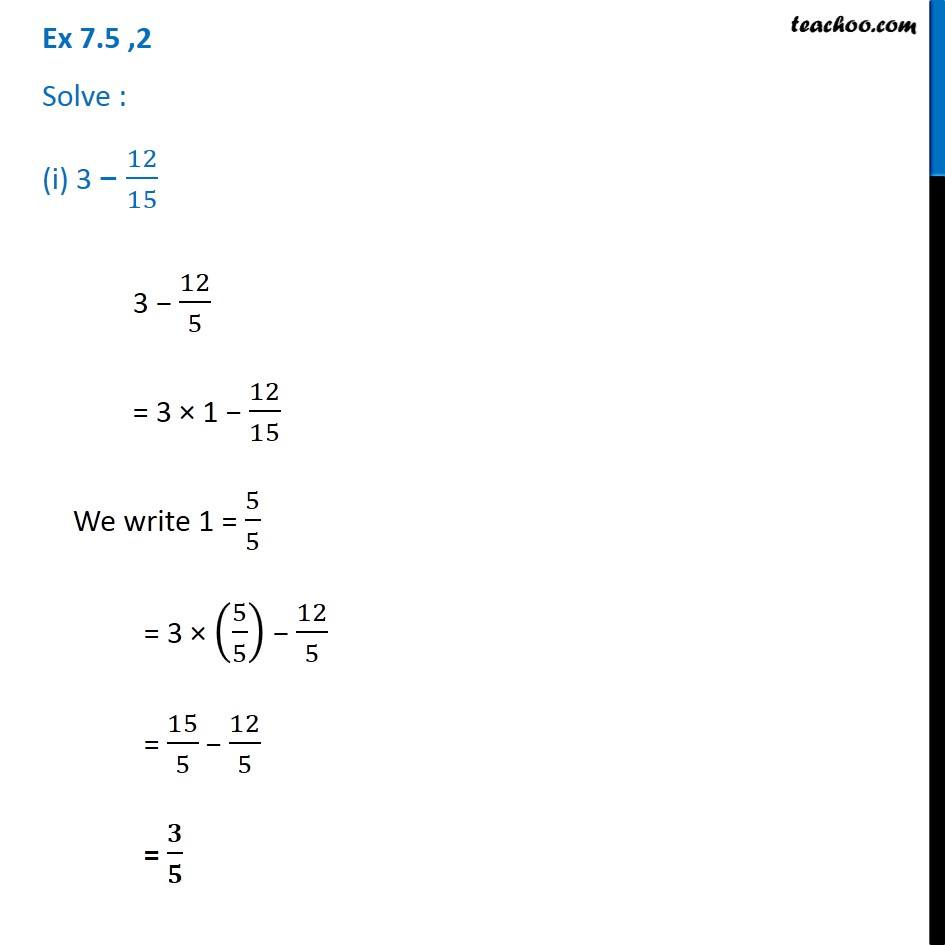Ex 7.5

Chapter 7 Class 6 Fractions
Serial order wiseGet live Maths 1-on-1 Classs - Class 6 to 12

### Transcript

Ex 7.5 ,2 Solve : (i) 3 − 12/153 − 12/5 = 3 × 1 − 12/15 We write 1 = 5/5 = 3 × (5/5) − 12/5 = 15/5 − 12/5 = 𝟑/𝟓 3 − 12/5 = 3 × 1 − 12/15 We write 1 = 5/5 = 3 × (5/5) − 12/5 = 15/5 − 12/5 = 𝟑/𝟓